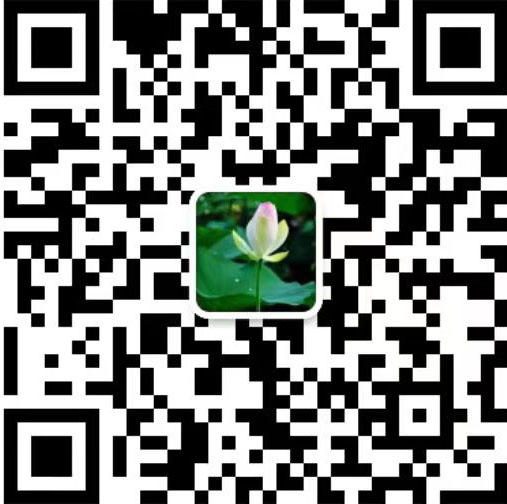## 25年超高压液压产品专业制造商集开发-设计 - 生产 - 销售于一体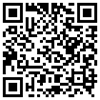手机网站

### 液压钳/线缆剪/钢筋剪系列

13901431334 （微信同号）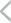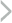•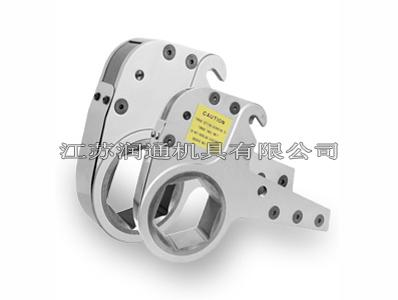NJZK / HSZK液压扳手工作头系列

1、配合多种不同规格的螺栓，提高适应性2、可定制特殊工作头配套设备：NJZK/HSZK系列中空扭矩扳手

13901431334（微信同号）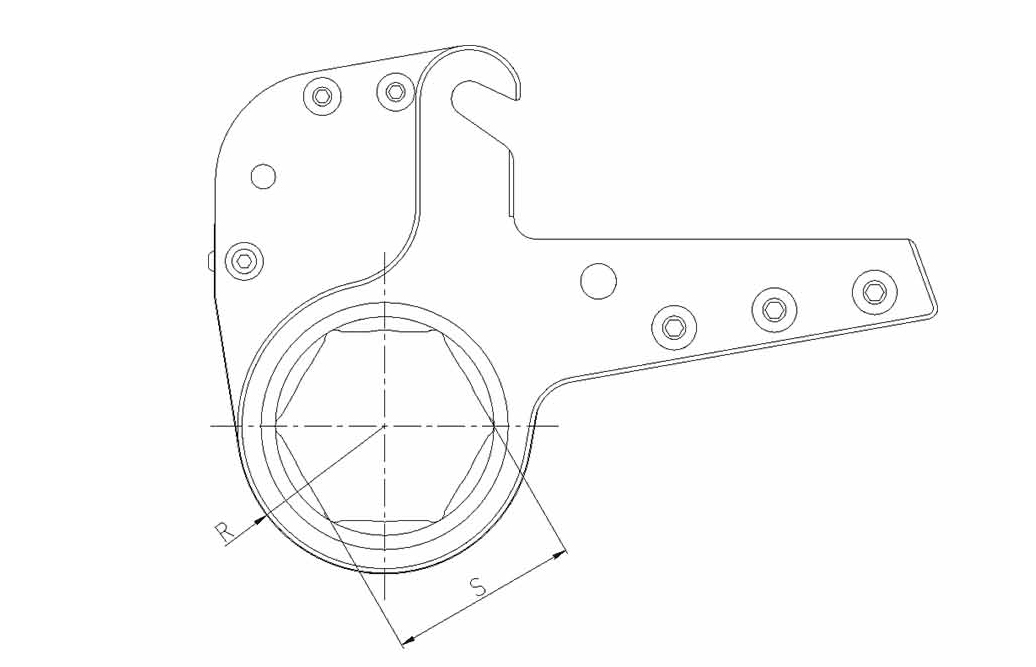扳手型号 工作头型号 工作头型号 螺栓 螺母对边 操作半径 2NJZK 2NJZK-36 M24 S=36 R=31 2NJZK-41 M27 S=41 R=34 2NJZK-46 M30 S=46 R=37 2NJZK-50 M33 S=50 R=40 2NJZK-55 M36 S=55 R=43 2NJZK-60 M39 S=60 R=46 4NJZK 4NJZK-46 M30 S=46 R=42 4NJZK-50 M33 S=50 R=44 4NJZK-55 M36 S=55 R=46 4NJZK-60 M39 S=60 R=50 4NJZK-65 M42 S=65 R=53 4NJZK-70 M45 S=70 R=56 4NJZK-75 M48 S=75 R=59 4NJZK-80 M52 S=80 R=62 8NJZK 8NJZK-70 M45 S=70 R=58 8NJZK-75 M48 S=75 R=60 8NJZK-80 M52 S=80 R=63 8NJZK85 M56 S=85 R=65 8NJZK-90 M60 S=90 R=68 8NJZK-95 M64 S=95 R=71 8NJZK-100 M68 S=100 R=75 8NJZK-105 M72 S=105 R=78 14NJZK 14NJZK-80 M52 S=80 R=67 14NJZK-85 M56 S=85 R=69 14NJZK-90 M60 S=90 R=72 14NJZK-95 M64 S=95 R=76 14NJZK-100 M68 S=100 R=78 14NJZK-105 M72 S=105 R=81 14NJZK-110 M76 S=110 R=85 14NJZK-115 M80 S=115 R=87

 扳手型号 工作头型号 工作头型号 螺栓 螺母对边 操作半径 18NJZK 18NJZK-85 M56 S=85 R=73 18NJZK-90 M60 S=90 R=75 18NJZK-95 M64 S=95 R=77 18NJZK-100 M68 S=100 R=77 18NJZK-105 M72 S=105 R=83 18NJZK-110 M76 S=110 R=83 18NJZK-115 M80 S=115 R=88 18NJZK-120 M85 S=120 R=93 20NJZK 20NJZK-90 M60 S=90 R=77 20NJZK-95 M64 S=95 R=80 20NJZK-100 M68 S=100 R=80 20NJZK-105 M72 S=105 R=85 20NJZK-110 M76 S=110 R=85 20NJZK-115 M80 S=115 R=90 20NJZK-120 M85 S=120 R=95 20NJZK-130 M90 S=130 R=102 30NJZK 30NJZK-95 M64 S=95 R=83 30NJZK-100 M68 S=100 R=83 30NJZK-105 M72 S=105 R=89 30NJZK-110 M76 S=110 R=89 30NJZK-115 M80 S=115 R=94 30NJZK-120 M85 S=120 R=98 30NJZK-130 M90 S=130 R=104 30NJZK-145 M100 S=145 R=114 30NJZK-150 M105 S=150 R=116 30NJZK-155 M110 S=155 R=116 30NJZK-165 M115 S=165 R=128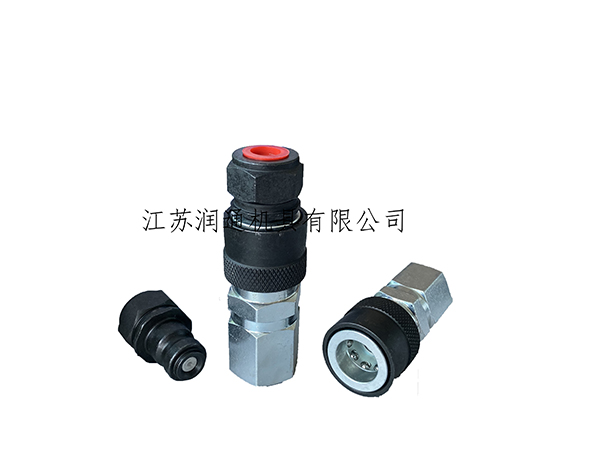### HS-140 140MPA液压快速接头（碳钢）(042)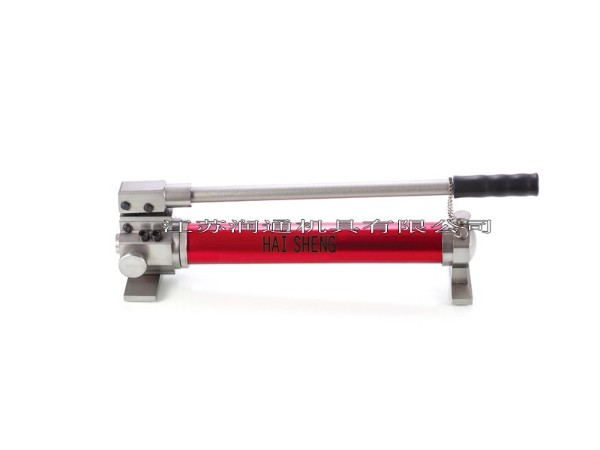### 新型全铝超轻手动泵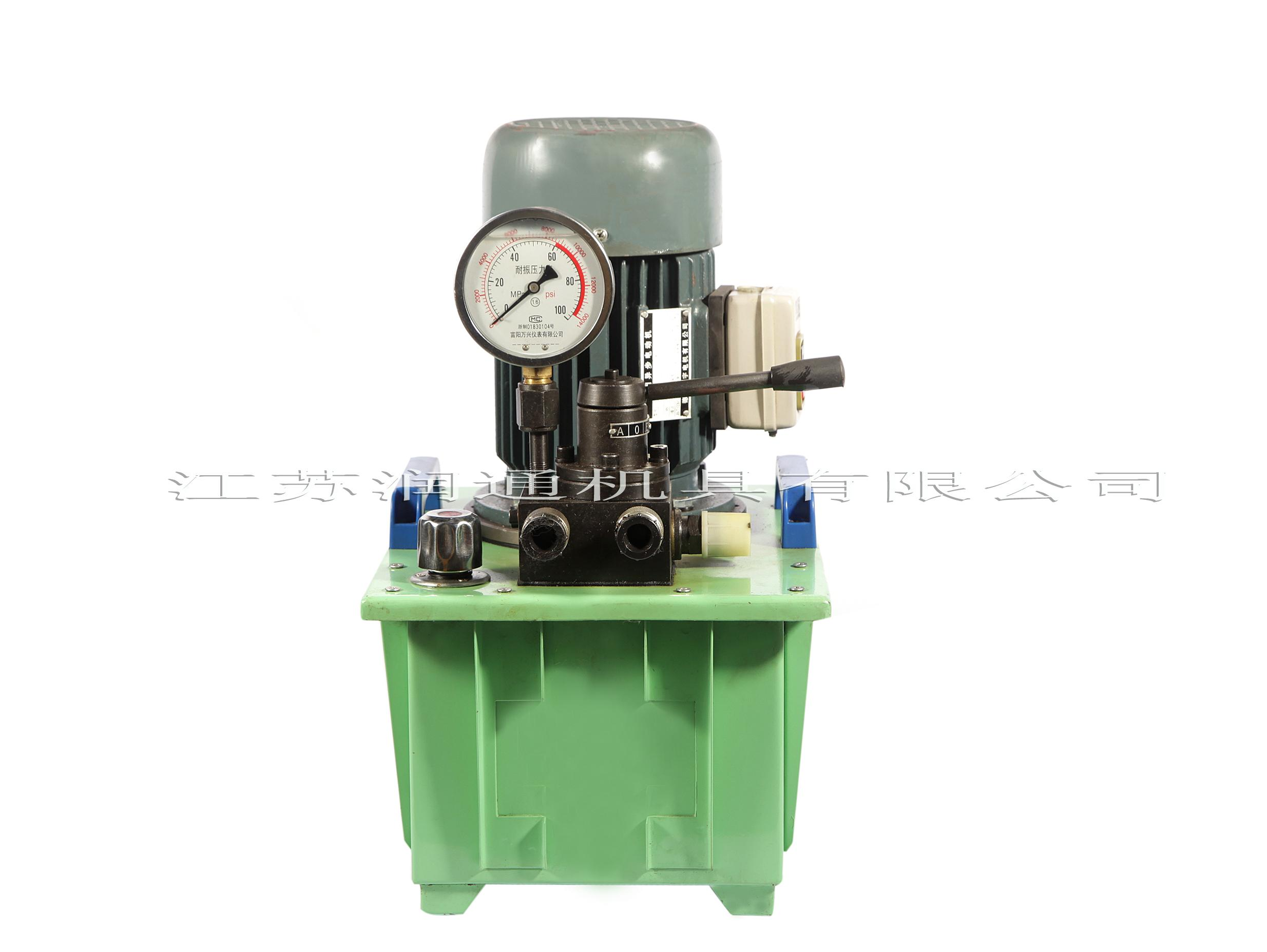### DBS系列液压电动泵

DBS系列电动泵站是由超高压柱塞泵、控制阀、电机、油箱、仪表等组成的一种独立，完整的液压动力装置。本泵为单级泵、双油路，配有手动换向阀。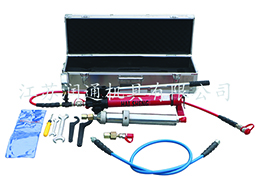### HS-35S手动试压注脂两用枪（2-35Mpa可调）

• 江苏润通机具有限公司 　备案号：苏ICP备16007854号
• 服务热线：13901431334（微信同号）　E-Mail：office@rtjiju.com
• 电话号码：13901431334（微信同号） 　传真：0523-86866902
•
•打开手机网站

• 电话

13901431334 （微信同号）
• 手机扫一扫